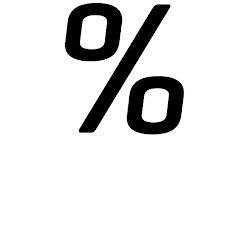# Square

If the length of the sides of the square we decrease by 25% decrease the content area of 28 cm2. Determine the side length of the original square.

Correct result:

a =  8 cm

#### Solution:

Our quadratic equation calculator calculates it.We would be pleased if you find an error in the word problem, spelling mistakes, or inaccuracies and send it to us. Thank you!Tips to related online calculators
Looking for help with calculating roots of a quadratic equation?
Do you have a linear equation or system of equations and looking for its solution? Or do you have quadratic equation?

#### You need to know the following knowledge to solve this word math problem:

We encourage you to watch this tutorial video on this math problem:

## Next similar math problems:

• Perimeter and legsDetermine the perimeter of a right triangle if the length of one leg is 75% length of the second leg and its content area is 24 cm2.
• Right triangleLegs of the right triangle are in the ratio a:b = 2:8. The hypotenuse has a length of 87 cm. Calculate the perimeter and area of the triangle.
• Pyramid cutWe cut the regular square pyramid with a parallel plane to the two parts (see figure). The volume of the smaller pyramid is 20% of the volume of the original one. The bottom of the base of the smaller pyramid has a content of 10 cm2. Find the area of the
• Square vs rectangleSquare and rectangle have the same area contents. The length of the rectangle is 9 greater and width 6 less than side of the square. Calculate the side of a square.
• Secret treasureScouts have a tent in the shape of a regular quadrilateral pyramid with a side of the base 4 m and a height of 3 m. Determine the radius r (and height h) of the container so that they can hide the largest possible treasure.
• Square sideIf we enlarge the square side a = 5m, its area will increase by 10,25%. How many percent will the side of the square increase? How many percent will it increase the circumference of the square?
• A rectangle 2A rectangle has a diagonal length of 74cm. Its side lengths are in ratio 5:3. Find its side lengths.
• TrapezoidArea of trapezoid is 135 cm2. Sides a, c and height h are in a ratio 6:4:3. How long are a,c and h? Make calculation...
• The ballThe ball has a radius of 2m. What percentage of the surface and volume is another sphere whose radius is 20% larger?
• Rectangle diagonalsIt is given rectangle with area 24 cm2 a circumference 20 cm. The length of one side is 2 cm larger than length of second side. Calculate the length of the diagonal. Length and width are yet expressed in natural numbers.
• AP RT triangleThe length of the sides of a right triangle form an arithmetic progression, longer leg is 24 cm long. What are the perimeter and area?
• Roof coverAbove the pavilion with a square ground plan with a side length of a = 12 m is a pyramid-shaped roof with a height v = 4.5 m. Calculate how much m2 of sheet metal is needed to cover this roof if 5.5% of the sheet we must add for joints and waste.
• BookshelveBookshelve with an original price of € 200 twice become cheaper. After the second discounted by 15% the price was € 149.60. Determine how much % become cheaper for the first time.
• Trapezoid 15Area of trapezoid is 266. What value is x if bases b1 is 2x-3, b2 is 2x+1 and height h is x+4
• Folding tableThe folding kitchen table has a rectangular shape with an area of 168dm2 (side and is 14 dm long). If necessary, it can be enlarged by sliding two semi-circular plates (at sides b). How much percent will the table area increase? The result round to one-hu
• Uboid volumeCalculate the cuboid volume if the walls are 30cm², 35cm², 42cm²
• Squares ratioThe first square has a side length of a = 6 cm. The second square has a circumference of 6 dm. Calculate the proportions of the perimeters and the proportions of the contents of these squares? (Write the ratio in the basic form). (Perimeter = 4 * a, conte